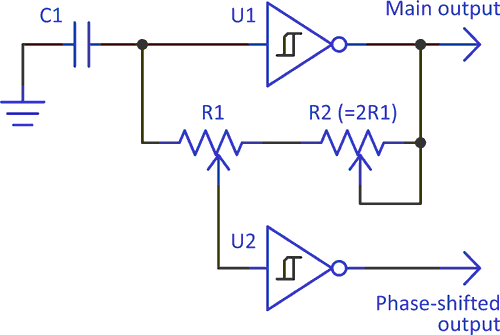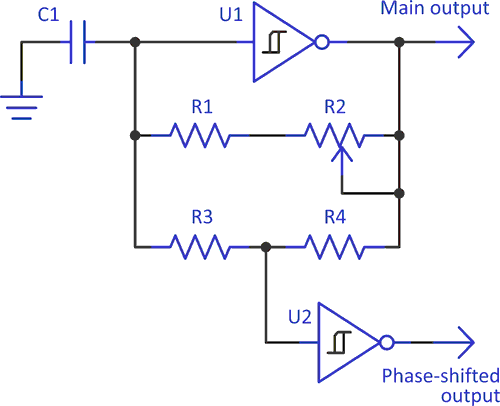# Add phases to simple RC oscillator

Einar Abell

EDN

This Design Idea demonstrates a simple way to generate a multiphase clock signal, the frequency of which can be varied with minimal change in phase shift(s).

The phase shift of the second output can be tuned from near zero to 180° without affecting the frequency. The basic circuit uses a minimum of parts: one cap, two resistors, plus two Schmitt triggers (Figure 1).Figure 1. Two-phase oscillator with adjustable frequency & phase-shift.

Frequency is a function of C1 and the sum of R1 and R2. U2 provides the phase shifted output – which leads the master output of U1 – via the tap on variable resistor R1. When the output of U1 is high and C1 is charging up, the voltage at the input to U2 will be higher than the voltage at the input to U1; therefore U2 will change state before U1, creating a phase lead. When U1’s output is low, the input to U2 will be lower than U1’s input, and it will again trigger ahead of U1. The closer the wiper of R1 is to R2, the greater the phase lead.

With CMOS Schmitt triggers, the input thresholds are not perfectly symmetrical relative to the output, and the two phase shifts will not be equal. This asymmetry is particularly noticeable with small phase leads.

R1 controls the phase shift, and R2 can be varied to trim the frequency (since this will alter phase, you should trim frequency, then phase). I show variable resistors in the schematic, but you can make one or both fixed; or, make R1 and R2 a single variable resistor, giving no frequency adjustment and a restricted adjustment range. Slight differences in the threshold voltages between U1 and U2 prevent setting the phase shift to zero or very close to it.

Figure 2 shows a way to vary frequency with minimal change in phase. In this circuit, R1 and R2 control frequency and R3 and R4 set the phase. The sum of R3 and R4 should be equal or greater than the sum of R1 and R2. You can achieve an adjustment range of a decade or so with minimal change in phase. Trying for more doesn't work too well because the input capacitance of U2 causes a phase lag, and this becomes significant at higher frequencies.Figure 2. Greater independence of frequency & phase.

Тo keep things simple, I show only a two phase circuit, but more phases are possible by adding more resistors or pots (a classic three-phase oscillator could be created from Figure 2 if R3 was split into equal halves and the signal from this midpoint was inverted relative to the other two phases). This idea is not limited to CMOS Schmitt triggers; it can also be used with oscillators made from comparators or a 556.

EDN